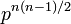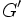# Upper bound on size of Schur multiplier for group of prime power order based on prime-base logarithm of order

Suppose$p$ is a prime number and$G$ is a finite p-group of order$p^n$. Then, the Schur multiplier of$G$ is also a finite$p$-group and its order is at most$p^{n(n-1)/2}$. In fact, we can say something stronger:$\! |G'||M(G)| \le p^{n(n-1)/2}$
where$G'$ denotes the derived subgroup of$G$.